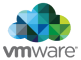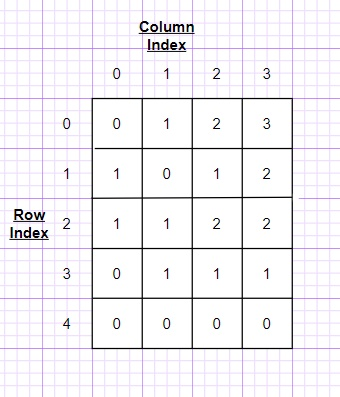New update is available. Click here to update.
Topics

# Fire in the cells.

Hard0/120
Average time to solve is 15m## Problem statement

You are given a matrix 'MAT' of size ‘N’ * ‘M’, where ‘N’ is the number of rows and ‘M’ is the number of columns. Value ‘0’ in a cell represents that the cell is set on fire initially, (at time t = ‘0’), and the cells which don’t have fire initially have value = ‘1’, and are called safe cells.

If a cell is on fire, then in one second the fire will expand to its adjacent cells (left, right, top, bottom) if they are not already on fire.

You are given the position of a person as integers ‘X’ and ‘Y’ denoting the cell (‘X’, ‘Y’). In one second the person can move from its current cell to any one of its adjacent cells, provided they are not on fire.

You have to determine if the person can move through the matrix to one of the escape cells without burning himself i.e. without going through the fire cells. If it’s possible, return time taken, else return -1.

Note:

``````1. Escape cells in this problem are all such cells that lie on the edge of the matrix but not on the corner. i.e all such cells which are in the first row, first column, last row, and last column excluding the four corner cells are considered as valid escape cells.

2. A cell once set on fire continues to remain on fire till the end.

3. Note that rows are indexed from 0 to ‘N’ - 1 and columns are indexed from 0 to ‘M’ - 1.

4. The escape cells may also be initially on fire or can catch fire from some neighboring cell.
``````
Detailed explanation ( Input/output format, Notes, Images )
Constraints:
``````1 <= 'T' <= 50
0 <= 'N' < 50
0 <= 'M' < 50
0 <= 'X' <= N
0 <= 'Y' <= M

Time Limit: 1 sec
``````
Sample Input 1:
``````1
5 4
0 1 1 1
1 0 1 1
1 1 1 1
0 1 1 1
0 0 0 0
2 1
``````
Sample Output 1:
``````-1
``````
Explanation For Sample Output 1:
``````The time for the fire to reach the individual cells of the given matrix is as follows:
````````````The person’s initial cell is (2, 1).
At time t = 1, the person can only go to cell (2, 2) as the fire won’t have reached the cell yet.
At time t = 2, the person can not go anywhere as all the adjacent cells are at the fire, and moving causes him to get burnt.
``````
Sample Input 2:
``````1
4 4
0 1 1 1
1 0 1 1
1 1 1 1
0 1 1 0
1 2
``````
Sample Output 2:
``````1
``````Console